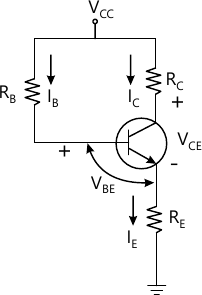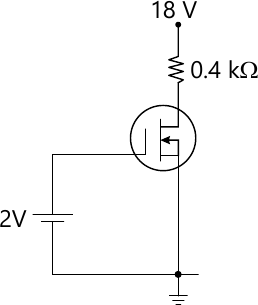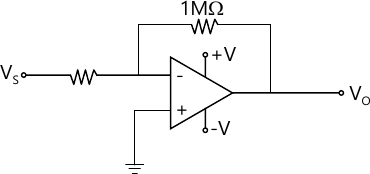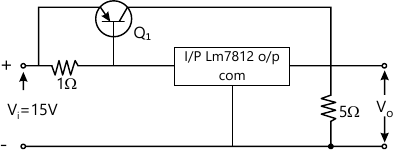MORE IN Analog and Digital Electronics
VTU Computer Science (Semester 3)
Analog and Digital Electronics
June 2013
Total marks: --
Total time: --
INSTRUCTIONS
(1) Assume appropriate data and state your reasons
(2) Marks are given to the right of every question
(3) Draw neat diagrams wherever necessary

1 (a) With neat figures, explain the construction and operational principle of an Uni Junction Transistor (UJT).
10 M
1 (b) Find the values of resistors RB, RC, RE and the transistor gain β, for the circuit shown in Fig.Q1(b). Given that, IB=40 μA, IC=4 mA, VE=2V, VCE=12 V and supply voltage VCC=15 V. Assume that the transistor used in the circuit is a silicon transistor.5 M
1 (c) Explain thermal runaway as referred to transistor.
5 M

2 (a) What are the differences between JFET's and MOSFET's?
5 M
2 (b) With the help of neat figures, explain the construction and characteristics of N-channel depletion MOSFET.
10 M
2 (c) Fig.Q2(c) shows a biasing configuration using DE-MOSFET. Given that the saturation drain current is 8 mA and the pinch off voltage is - 2 V. Determine the value of gate-source voltage, drain current and the drain source voltage.5 M

3 (a) Define the following terms:
(i) Responsivity (R)
(ii) Noise equivalent power (NEP)
(iii) Detectivity
(iv) Quantum efficiency
(v) Response time.
5 M
3 (b) What is photo transistor? Draw the schematic symbol of a phototransistor. Explain the V-I characteristics of photo transistor.
5 M
3 (c) Explain different modes of operation of an LCD display.
10 M

4 (a) Draw the generalized h-parameter model of a transistor based amplifier and derive the expression for:
(i) Current gain
(ii) Input impedance
(iii) Voltage gain
10 M
4 (b) With neat figure explain the operation of Darlington amplifier.
5 M
5 M

5 (a) What are the advantages of negative feedback?
5 M
5 (b) Derive the relevant expressions to prove that input resistance increases and output resistance reduces in case of voltage-series feed back.
8 M
5 (c) Refer to Fig.Q5(c) of op-amp based inverting amplifier circuit. Identity the type of negative feedback. Determine the trans impedacnce gain, the input impedance and output impedance of the amplifier, given that transimpedance, input impedance and output impedance parameters of the op-amp are 100 MΩ, 10 MΩ adn 100 Ω respectively.7 M

6 (a) Explain the Bark hausen criterion as referred to oscillators.
5 M
6 (b) With neat figure, explain the opration of voltage controlled oscillator.
7 M
6 (c) With neat figure and relevant wave forms explain the operation of astable multivibrator using IC555 timer.
8 M

7 (a) Name the constituent parts of a basic linearly regulated power supply. Briefly describe the function of each of the constituent parts.
5 M
7 (b) Define: (i) load regulation (ii) line regulation (iii) ripple rejection factor with reference to regulated power supplies.
3 M
7 (c) With neat figure, explain the working of buck-regulator.
7 M
7 (d) Refer to the three terminal regulator circuit of Fig.Q7(d),Determine: (i) Load current (ii) Current through LM7812 (iii) Current through external transistor dissipated in LM7812. Take VBE(Q1)=0.7V.5 M

8 (a) Define the following as referred to op-amp (i) CMRR (ii) PSRR (iii) Slew rate (iv) Band width (v) Open loop gain
5 M
8 (b) With neat figure, explain the opration of a peak detector circuit using op-amp.
7 M
8 (c) With neat figure and relevant wave forms explain the working of relaxation oscillator circuit using op-amp.
8 M

More question papers from Analog and Digital Electronics